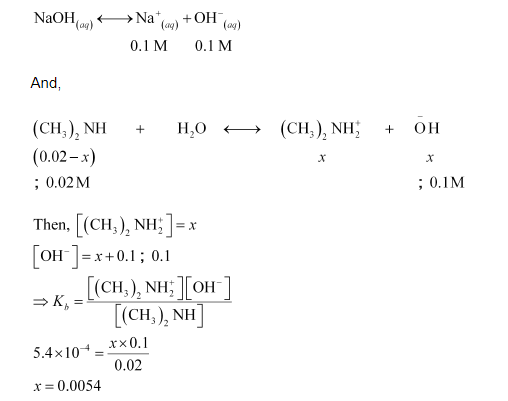# The ionization constant of dimethylamine is 5.4 × 10–4. Calculate its degree of ionization in its 0.02 M solution

Question:

The ionization constant of dimethylamine is $5.4 \times 10^{-4}$. Calculate its degree of ionization in its $0.02 \mathrm{M}$ solution. What percentage of dimethylamine is ionized if the solution is also $0.1 \mathrm{M}$ in $\mathrm{NaOH}$ ?

Solution:

$K_{b}=5.4 \times 10^{-4}$

$c=0.02 \mathrm{M}$

Then, $\alpha=\sqrt{\frac{K_{b}}{c}}$

$=\sqrt{\frac{5.4 \times 10^{-4}}{0.02}}$

$=0.1643$

Now, if 0.1 M of NaOH is added to the solution, then NaOH (being a strong base) undergoes complete ionization.It means that in the presence of $0.1 \mathrm{M} \mathrm{NaOH}, 0.54 \%$ of dimethylamine will get dissociated.